# Evolution of the Complex Motor Reaction Test Results for Children Practicing Football

## Abstract

The training process, oriented toward the stimulation of children to get involved in solving independently various unexpectedly-occurred game situations and created by the coach on purpose, is an indispensable operation, but is also considered a stimulus for high performance. Preparing children by using problem solving in training needs to be approached systematically, taking into account the benefits that can be found in the development of productive and independent thinking; this method develops operation schemes of the divergent thinking, as well as its expression through quick decisions and accurate motor reactions in solving the problematic situations occurred. This study aims to highlight the improvement of motor reaction in football players according to their technical level, as a result of using in training an operational program designed in compliance with the principles of the question method. The operational program was applied for 8 months to a sample of 20 players aged 11 to 12 years. The evolution of using the question method was assessed by the Complex Motor Reaction Test applied at the beginning and the end of the program. The experimental research was conducted on the sports ground of “Mircea Eliade” Sports High School and at the Psychopedagogy Laboratory of the National University of Physical Education and Sports (UNEFS), Bucharest. The assimilation of knowledge by deduction, discovery and divergent thinking has revealed dynamic learning and solid knowledge, the processes of information storage and awareness being carried out more attractively.

Keywords: Motor reactionproblem solvingtechnical trainingfootball

## Introduction

Our scientific approach starts from the premise that football training in children and juniors is mostly focused on explanatory exemplifying methods related to the coach’s communication of acknowledged information and pre-established solutions verified over time, which lead in particular to the development of memory and convergent thinking.

Due to the fact that football has a situational character, in order to make the training more efficient, it is necessary to use an operational program oriented toward the principles of the problem-solving method, which involves the creation of complex theoretical and practical situations requiring the players to concentrate on finding optimal solutions, in accordance with the unpredictable nature of the moments of the game, but especially with the short solving time that requires quick thinking and decision.

The design of a training program based on problem solving involves developing, planning, experimenting and validating an educational study in the true meaning of the word, but also a pretentious goal, because in practice this requires creativity, fantasy, concern and inventiveness from the coach. He must have the ability to create problem situations in which real aspects of the game can be found so as to make the player select the optimal solution for the purpose of developing his creativity and reaction.

With regard to the training process, encouraging children to engage in the independent solving of various emerging or intentional situations is an indispensable operating way, which also stimulates them to achieve great performance and not only.

This pedagogical process, which is mainly focused on using the problem-solving method, proposes a modern vision of training with the intention to make a significant contribution for increasing the players’ performance by designing a scientifically-guided training program, in which both its content and scheduling are performed in accordance with the current and future norms for the development and improvement of children and juniors.

## Problem statement

Using the problem-solving method in the training of football players aged 11-12 years is a common issue nowadays, taking into account the training of young footballers in accordance with the situational problems encountered by them during the game.

As Nicola (1994) considered, “this method contains a series of procedures that aim to create problem situations which prepare for and give students or learners the opportunity to observe different relationships between objects and phenomena of reality” (p. 104). At the same time, David (2001) characterizes problem solving as “the most heuristic and activating method” (p. 158). This incites the athlete toward interest and involvement, by using memory and speed of analysis and decision. Jinga (2001) claims that “the problem situation is a surprise factor, even a novelty with which the subject is put in contact” (p. 117), but also a factor requiring creative thinking.

Raţă (2008) thinks a method frequently encountered in performance sport, mainly in sports games ” it requires the creation of problem situations that require the athletes’ special attention in finding the most effective solutions”, but also “practice by means of deduction and creative thinking” (p. 73).

## Research questions

By analyzing the theoreticians’ opinion regarding the use of problem-solving method in training 11-12 years old juniors, the following questions arise:

• Does problem solving as a method have implications on the players’ overall performances in the official games and also on their proper development as footballers?

• Is there a connection between the application of problem solving as a training method and the improvement of motor reaction in junior football players aged 11-12 years?

• Is the tactical level of players shown in training and official games influenced by the use of problem solving as an operational program, and in what way?

## Purpose of the study

The present study aims at highlighting the use of the problem-solving method by applying an operational program in the training process, with effect on improving motor reaction of the football players, in accordance with their technical-tactical level.

## Research methods

For the experimental research, we applied the following methods: bibliographic study, pedagogical observation, evaluation method, experimental method, testing, statistical-mathematical method and graphical method.

This research has as a starting point the hypothesis that performing twice a week some action systems designed according to the problem-solving method leads (with three training sessions/week) to the improvement of motor reaction in football players aged 11-12 years. Their parents agreed to preadolescents’ participation in the study.

Between 15 September 2016 and 15 April 2017, we carried out the evaluation activity through the initial and final testing in the Psychopedagogy Laboratory of UNEFS Bucharest. The evolution of using the problem-solving method was assessed by the Complex Motor Reaction Test 1 (CMRT 1) applied to a sample of 20 players aged 11 to 12 years, belonging to the FCSB Football Club.

The operational program was applied for 8 months to the previously mentioned sample, on the sports ground of “Mircea Eliade” Sports High School. This operational program comprised a number of 48 action systems designed according to the problem-based method and structured in four categories: movement games, individual techniques, situational relations of adversity (1 vs. 1, 2 vs. 1, 2 vs. 2, 3 vs. 3, 4 vs. 3, 4 vs. 4, 5 vs. 4, 5 vs. 5) and technical-tactical ones.

The action systems were used in two of the three training sessions of the week, three times each in the training session.

## Findings

The 20 children from the FCSB Football Club performed the Psychometric Test – Complex Motor Reaction both in the initial and the final testing, following the evolution of 8 coefficients within the test.

The descriptive results and the statistical significance scores of the differences between group averages in the initial and final testing for the 8 psychometric test coefficients – Complex Motor Reaction Test are presented in Table 01 .

The results for the learning ability coefficient fall between 140 and 923 in the initial test, with an average of 513.40, and between 182 and 998 in the final test, with an average of 714.60 (Table 01 and Figure 01 ). The evolution of this coefficient (an average increase of 201.20) is statistically significant, the p-value being less than 0.05 (by applying the Student dependent test). The calculated t-value is -8.208, and p = 0.000.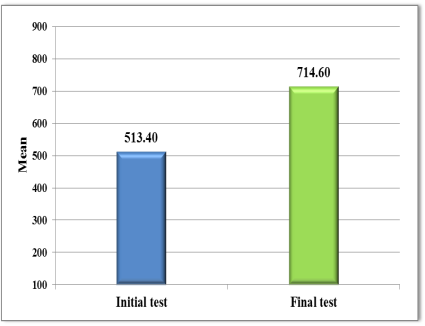Figure 1: Initial testing vs. final testing for the learning ability coefficient

The results for the operating memory coefficient fall between 772 and 983 in the initial testing, with an average of 874.45, and between 756 and 999 in the final testing, with an average of 944.60 (Table 01 and Figure 02 ). The evolution of this coefficient (an average increase of 70.15) is statistically significant, the p-value being less than 0.05 (by applying the dependent Student test). The calculated t-value is -3.374, and p = 0.003.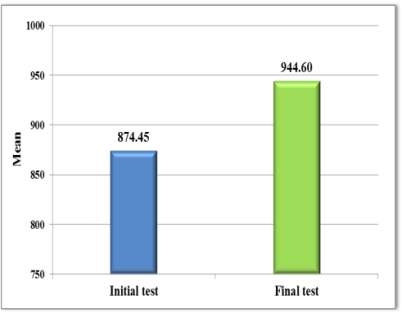Figure 2: Initial testing vs. final testing for the operating memory coefficient

The results for the sample performance coefficient fall between 301 and 597 in the initial testing, with an average of 453.20, and between 318 and 712 in the final testing, with an average of 484.50 (Table 01 and Figure 03 ). The evolution of this coefficient (an average increase of 14.65) is statistically insignificant, the p-value being greater than 0.05 (by applying the dependent Student test). The calculated t-value is -1.879, and p = 0.076.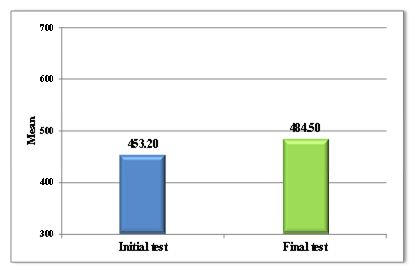Figure 3: Initial testing vs. final testing for the sample performance coefficient

The results for the time reaction to complex stimuli coefficient fall between 936 and 1456 in the initial testing, with an average of 1213.50, and between 904 and 1448 in the final testing, with an average of 1038.90 (Table 01 and Figure 04 ). The evolution of this coefficient (an average decrease of 174.60) is statistically significant, the p-value being less than 0.05 (by applying the dependent Student test). The calculated t-value is 5.127, and p = 0.000.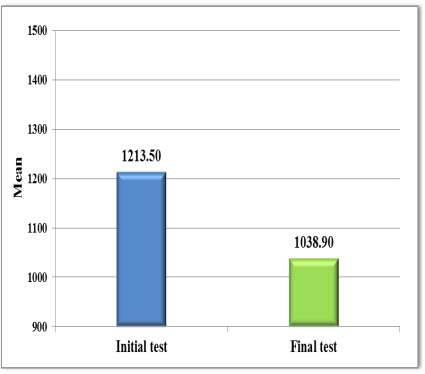Figure 4: Initial testing vs. final testing for the time reaction to complex stimuli coefficient

The results for the disturbing factor resistance coefficient fall between 814 and 1245 in the initial testing, with an average of 1048.35, and between 829 and 1253 in the final testing, with an average of 1054.30 (Table 01 and Figure 05 ). The evolution of this coefficient (an average increase of 5.95) is statistically insignificant, the p-value being greater than 0.05 (by applying the dependent Student test). The calculated t-value is -0.839, and p = 0.412.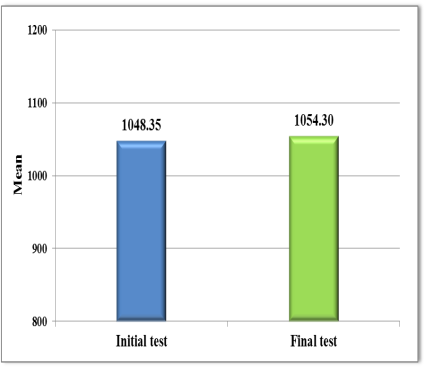Figure 5: Initial testing vs. final testing for the disturbing factor resistance coefficient

The results for the optimum personal rhythm coefficient fall between 26 and 474 in the initial testing, with an average of 161.85, and between 23 and 483 in the final testing, with an average of 162.95 (Table 01 and Figure 06 ). The evolution of this coefficient (an average increase of 1.10) is statistically insignificant, the p-value being greater than 0.05 (by applying the dependent Student test). The calculated t-value is -0.232, and p = 0.819.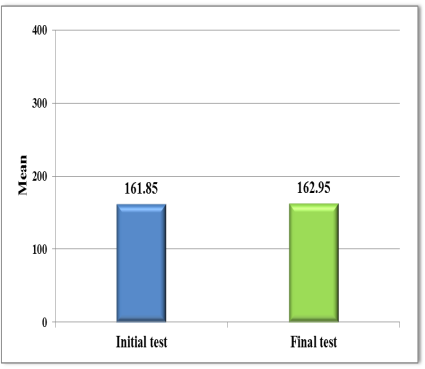Figure 6: Initial testing vs. final testing for the optimum personal rhythm coefficient

The results for the time-pressure resistance coefficient fall between 632 and 1060 in the initial testing, with an average of 813.45, and between 703 and 1285 in the final testing, with an average of 994.05 (Table 01 and Figure 07 ). The evolution of this coefficient (an average increase of 180.60) is statistically significant, the p-value being less than 0.05 (by applying the dependent Student test). The calculated t-value is - 5.098, and p = 0.000.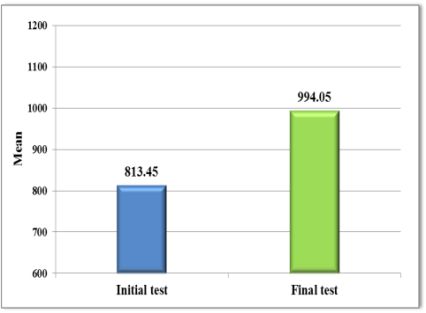Figure 7: Initial testing vs. final testing for the time-pressure resistance coefficient

The results for the self-pacing coefficient fall between 33.95 and 61.28 in the initial testing, with an average of 46.47, and between 33.28 and 56.77 in the final testing, with an average of 42.24 (Table 01 and Figure 08 ). The evolution of this coefficient (an average decrease of 4.23) is statistically significant, the p-value being less than 0.05 (by applying the dependent Student test). The calculated t-value is 4.137, and p = 0.001.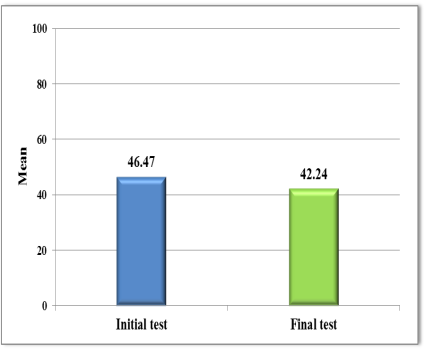Figure 8: Initial testing vs. final testing for the self-pacing coefficient

## Conclusions

From the analysis and interpretation of the results, we concluded that the development level of motor reaction in children aged 11-12 years has significantly improved after applying the operational program designed according to the possibilities of solving the problematic situations invented or emerged during training and based on the players’ special abilities at this age, in line with the challenges induced by the problem solving.

Analysing the obtained results, we found statistically significant improvements in 5 of the 8 evaluated coefficients, the p-value (by applying the dependent Student test) being less than 0.05 in all 5 cases:

• learning ability coefficient: calculated t-value = -8.208, p <0.05;

• operating memory coefficient: calculated t-value = -3.374, p <0.05;

• reaction time to complex stimuli: calculated t-value = 5.127, p <0.05;

• time-pressure resistance coefficient: calculated t-value = -5.098, p <0.05;

• self-pacing coefficient: calculated t-value = 4.137, p <0.05.

In 3 of the 8 evaluated coefficients, the 20 participants did not have a statistically significant evolution between the two tests, the p-value (by applying the dependent Student test) being greater than 0.05:

• sample performance coefficient: calculated t-value = -1.879, p> 0.05;

• disturbing factor performance coefficient: calculated t-value = -0.839, p> 0.05;

• optimum personal rhythm: calculated t-value = -0.232, p> 0.05.

Therefore, the entire scientific approach falls within the logic of education and training for children and juniors in order to improve their performance level regarding the physical, motor and mental training. After applying the research methods, performing the initial and final testing and analysing the data, the hypothesis according to which performing twice a week some action systems designed according to the problem-solving method leads (with three training sessions/week) to the improvement of motor reaction in football players aged 11-12 years, has been confirmed.

After conducting a study based on the initiation and motor reaction in the professional training process, Pavel (2015) emphasizes that “the motor level of balance and the movement control ability improve according to the development of the instructive-educational process” (p. 167), which highlights the importance of the educational practice.

The assimilation of knowledge by deduction, discovery and divergent thinking has highlighted dynamic learning and solid knowledge, the processes of information storage and awareness being carried out more attractively, while stimulating the young athletes to think and make a quick technical decision.

## References

05 March 2018

#### Article Doi

https://doi.org/10.15405/epsbs.2018.03.34

#### eBook ISBN

978-1-80296-035-8

36

-

1st Edition

1-484

#### Subjects

Sports, sport science, physical education, health psychology